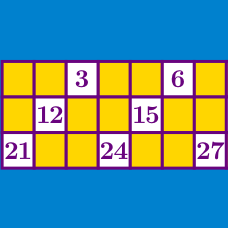Number Theory

# Even and Odd Numbers Warmup

$\large \text{Is } 0 \text{ even or odd?}$

What is true of all even numbers?

Given an integer $k$, which of these might be odd?

What is the $28 ^{\mbox{th}}$ smallest positive odd number?

What is true of all odd numbers?

×